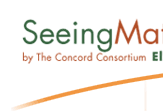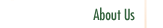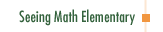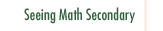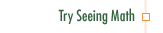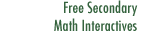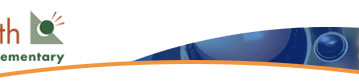Seeing Math™ Elementary: Dynamic Interactive Software Makes Math Come to Life

Seeing Math™ Elementary features dynamic interactive software that:

• Illustrates and reinforces key mathematical ideas.
• Accelerates learning and enhances comprehension of difficult concepts.

Broken Calculator Activity
The course Number & Operations: Broken Calculator examines how broken calculator problems can help students develop computational fluency. Faced with the challenge of solving problems without using certain disabled keys on their calculators, students must think creatively and use a variety of skills. They use number facts and estimation; they break numbers apart and recombine them; they use alternative operations to work around "broken" keys. They show each other how to use "friendly" numbers to approximate answers, and then figure out how to compensate to get the exact solution. Some problems require that students break multiplication problems into component parts and understand in detail how multi-digit multiplication works.

This four-part activity includes interactive software that enables participants to:

• Disable certain number or function keys on the calculator
• Set "goals"—either target numbers to reach or problems to solve
• Maintain a history of steps taken to solve problems.

Click to try the Broken Calculator Interactive.Course Listing Effective Questioning in the Mathematics Classroom Formative Assessment in the Mathematics Classroom Number & Operations: Division with Remainders Number & Operations: The Magnitude of Fractions Number & Operations: Broken Calculator Geometry: 2D and 3D Figures Geometry: Calculating Area of a Triangle Data Analysis and Probability: Using Data to Make Predictions Data Analysis and Probability: Measures of Center Pre-Algebra: Pan Balance Equations Pre-Algebra: Patterns and Functions